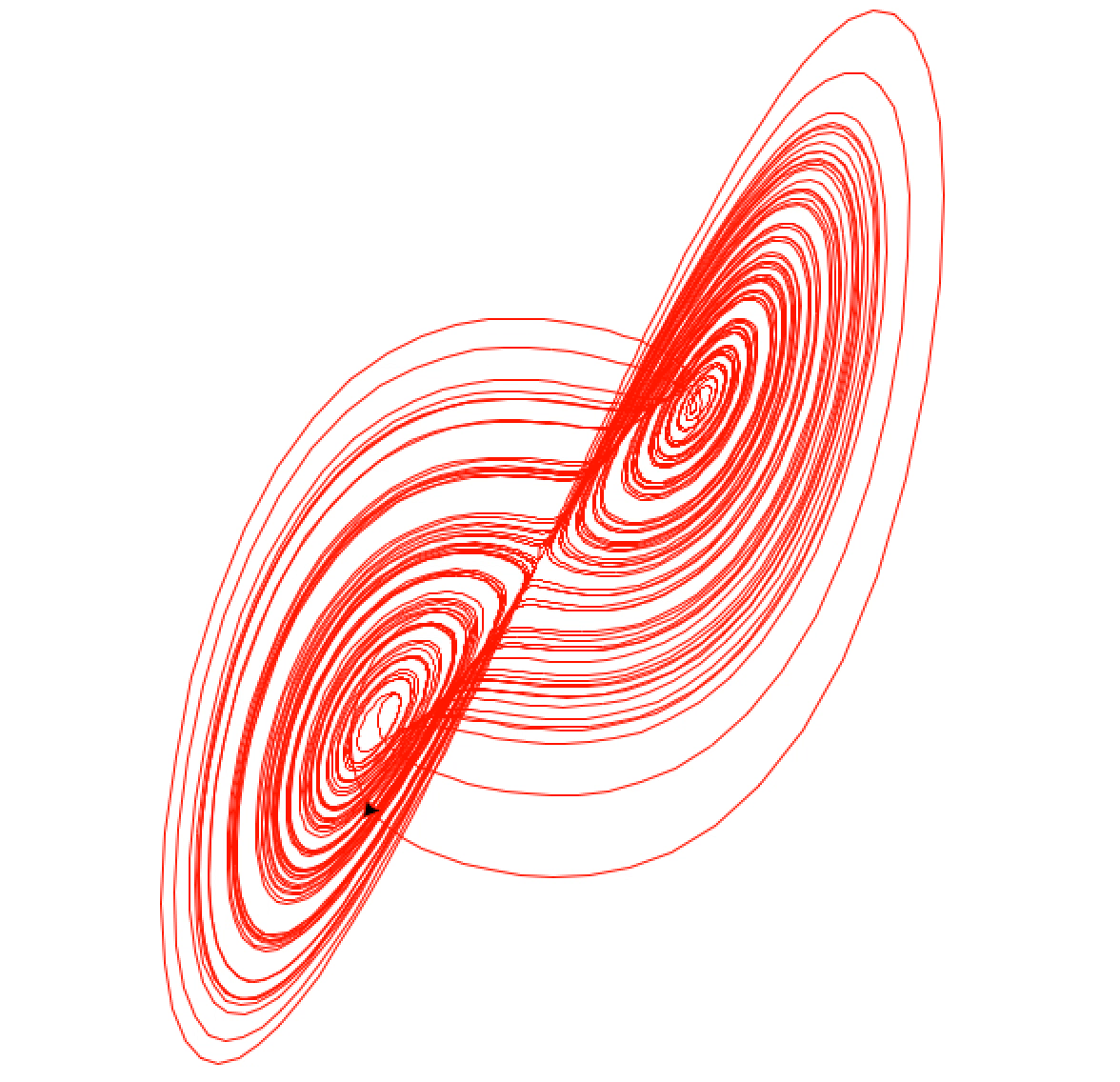# Lorenz attractor using turtle

I recently discovered that a nice way to visualize the behavior of the Lorenz system is to use Python’s turtle module. In case you didn’t know, the Lorenz system is defined by the equations:

\begin{align} \frac{dx}{dt} &= \sigma (y-x), \\ \frac{dy}{dt} &= x (\rho - z) - y, \\ \frac{dz}{dt} &= xy - \beta z. \end{align}

It’s interesting that such a simple system of differential equations can lead to such nontrivial results. Here’s what I came up with:

Pretty self-explanatory I think, but its output is pretty cool:Here is what gets produced after it runs for a while: